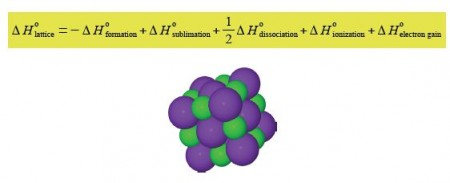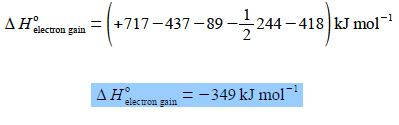# What is the Strength of an Ionic Crystal Structure?

An ionic crystal MX with M being the metal cation and X the non-metal anion (below figure) has a certain amount of intrinsic strength that holds the crystal structure together. This is called the lattice enthalpy ΔH0lattice, and is given by the equation,a small section of a KCI crystal, K+ = purple, CI = green.

The other physical quantities in the equation are for the present not important, although you will come across them early on in your Chemistry degree. Given the following data for potassium chloride crystals rearrange the above equation and calculate the enthalpy of electron gain ΔH0electron gain for the Cl anion.Solution

ΔH0lattice = ΔH0formation + ΔH0sublimation + ΔH0dissociation + ΔH0ionization + ΔH0electron gain

The equation is rearranged to give the electron gain enthalpy as the subject of the equation.

ΔH0electron gain = ΔH0lattice – ΔH0formation – ΔH0sublimation – ΔH0dissociation – ΔH0ionization

Substituting the physical quantities for the symbols and splitting the equation over two lines for clarity gives,

ΔH0electron gain = (+ 717 KJ mol-1) + (- 437 KJ mol-1) – (+ 89 KJ mol-1) – ½ (+ 244 KJ mol-1) + (+ 418 KJ mol-1)

Notice the difference between the mathematical operations of addition or subtraction as shown by plus or minus signs outside the brackets; and the positive or negative values of the physical quantities themselves shown by plus or minus inside the brackets. We can clean up the equation by removing the brackets and taking the common units out in  terms of ionic crystal structure.